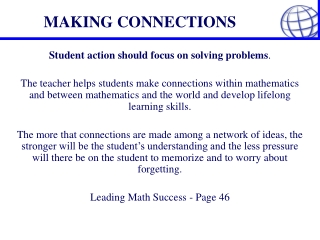DownloadDownload PresentationMAKING CONNECTIONS

# MAKING CONNECTIONS

Download Presentation## MAKING CONNECTIONS

- - - - - - - - - - - - - - - - - - - - - - - - - - - E N D - - - - - - - - - - - - - - - - - - - - - - - - - - -
##### Presentation Transcript

1. MAKING CONNECTIONS Student action should focus on solving problems. The teacher helps students make connections within mathematics and between mathematics and the world and develop lifelong learning skills. The more that connections are made among a network of ideas, the stronger will be the student’s understanding and the less pressure will there be on the student to memorize and to worry about forgetting. Leading Math Success - Page 46

2. A Rich Learning Task • On your table is a large sheet of paper. It holds a learning task, plus a place for reflecting on the six revision goals. • We will be coming back to the six revision goals throughout the next two days. On with the task!!

3. MAKING CONNECTIONS • The first three terms of a sequence are modeled in the picture below. • Create physical models for these 3 terms and the next 2 terms in this sequence for a total of 5 terms.

4. MATHEMATICAL MODELS Graphical Model Numerical Model

5. N = 2(n-1) + 1 N = (n - 1) + n N = n2 - (n - 1)2 MATHEMATICAL MODELS Algebraic Models

6. MATHEMATICAL MODELS Graphical Model Numerical Model ? Physical Model Algebraic Model N = 2n - 1

7. T = 2(n-1) + 1 T = (n - 1) + n T = n2 - (n - 1)2 MATHEMATICAL MODELS Algebraic Models T = 2n - 1

8. MATHEMATICAL MODELS Numerical Model Graphical Model ? Physical Model Algebraic Model T = 2n - 1

9. RICH LEARNING TASKS An extension to this problem: Which model (algebraic, numerical, etc.) would you use to determine the total number of cubes needed to make the first 50 terms? * Discuss your choice with a neighbor.

10. MATHEMATICAL MODELS It takes 52 or 25 cubes to make the first 5 terms so it takes 502 or 2500 cubes to make 50 terms.

11. RICH LEARNING TASKS Other extensions to this problem: How would this problem change if: • They started with a \$5 donation? • They charged \$2 per car? • One student charged \$1 and the other charged \$2? • … and so on.

12. RICH LEARNING TASKS • A problem solving approach encourages students to reason their way to a solution or a new understanding…. • The communication and reflection that occurs during and after the process of problem solving helps students not only to articulate and refine their thinking but also to see the problem they are solving from different perspectives. Draft Introduction Mathematics 9 and 10, 2005

13. LEARNING TASKS • “When developing detailed courses of study from this document, teachers are expected to weave together related expectations from different strands…” • “Problem solving is central to learning mathematics.” • “A balanced mathematics program at the secondary level includes the development of algebraic skills.” Draft Introduction: Curriculum Document 2005